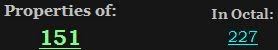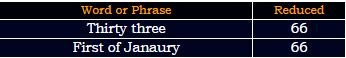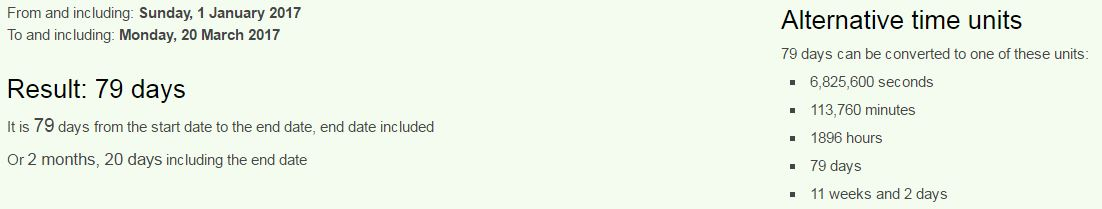## Cipher## Mar 21, 2017

### CNN - 33 incidents in 2017 - 33/Pi tributes on a 33 date numerology

CNN's story -Today is March 20th, a date with 33 numerology and CNN released a story giving a lot of tributes to 33, there is also some interesting Pi coding in this article starting from the time of release 22:07

The first sentence has 227 gematria of course
A mosque in Ypsilanti Michigan is burned to the ground = 1 + 4+6+1+8+3+5 + 9+5 + 7+7+1+9+3+1+5+2+9 + 4+9+3+8+9+7+1+5 + 9+1 + 2+3+9+5+5+4 + 2+6 + 2+8+5 + 7+9+6+3+5+4 = 227 (Reduced)2nd sentence sums to 364, much like a full year/full circleRoseville California = 9+6+1+5+4+9+3+3+5 + 3+1+3+9+6+6+9+5+9+1 = 97 (Reduced)
25th prime number is 97 (Pi = 25)
threats, vandalism or arson = 2+8+9+5+1+2+1 + 4+1+5+4+1+3+9+1+4 + 6+9 + 1+9+1+6+5 = 97 (Reduced)
incidents = 9+14+3+9+4+5+14+20+19 = 97 (Ordinal)
March = 4+1+9+3+8 = 25 (Reduced)
Pi = 16+9 = 25 (Ordinal)

Council on American-Islamic Relations = 3+6+3+5+3+9+3+6+5+1+4+5+9+9+3+1+5+9+1+3+1+4+9+3+9+5+3+1+2+9+6+5+1 = 151 (Reduced)Florida to Hawaii = 6+12+15+18+9+4+1 + 20+15 + 8+1+23+1+9+9 = 151 (Ordinal)

3/20/2017 - 3+20+2+0+1+7 = 33
They already made sure to highlight certain things for us, like 33 incidents from Janaury 1st to March 20thThere is 79 days from January 1st to the release of this article (22nd prime number is 79), also it is 2 months and 20 days a lot like 22 again, which connects to the circle and 22/7It is also 11 weeks and 2 days - Circle = 24+18+9+24+15+22 = 112 (Reverse Ordinal)

They say that in 2016 there were 17 incidents in the same time period
33-17 = 16 - Sixteen = 1+9+6+2+5+5+5 = 33 (Reduced)
Pi = 7+9 = 16 (Reduced)

The incidents, tallied by the Council on American-Islamic Relations, span 19 states from Florida to Hawaii.

Hawaii = 8+1+5+1+9+9 = 33 (Reduced)Admission to union on 3/3? 27 is 33 in octal system as well

Below the text they have listed 33 places where those "incidents" happened and the last one on the list just happens to sum to 777
Bellevue = 2+5+30+30+5+400+300+5 = 777 (English)